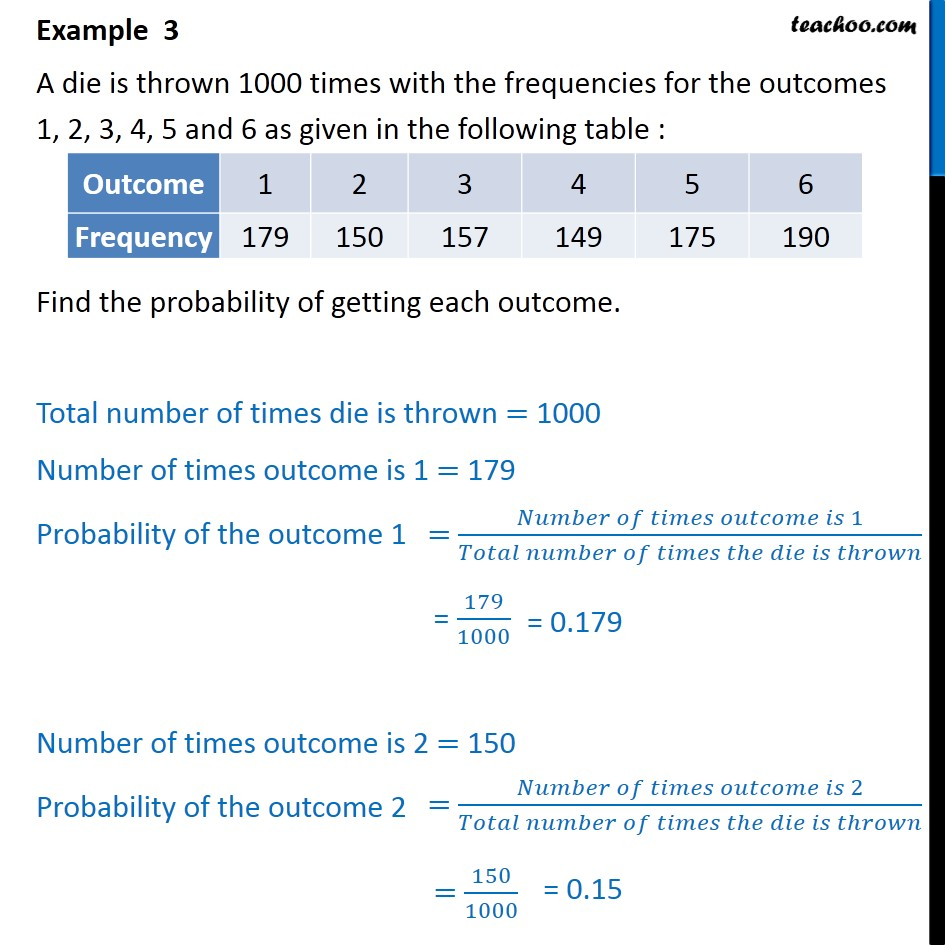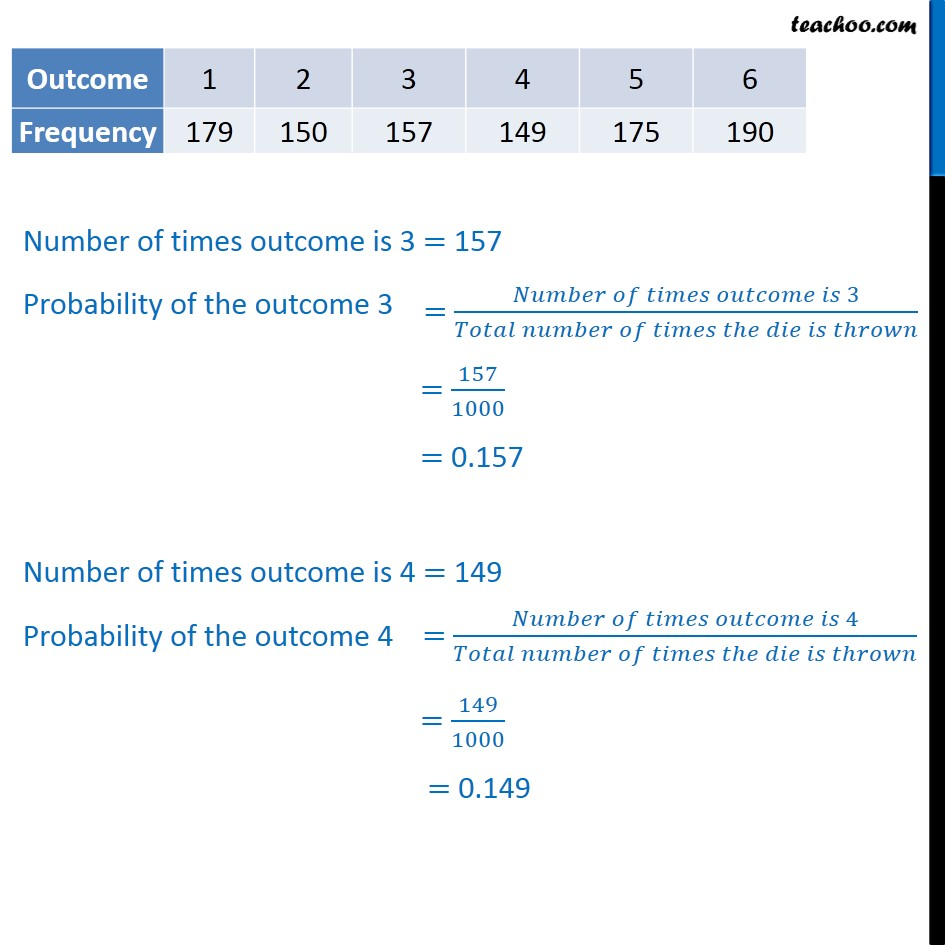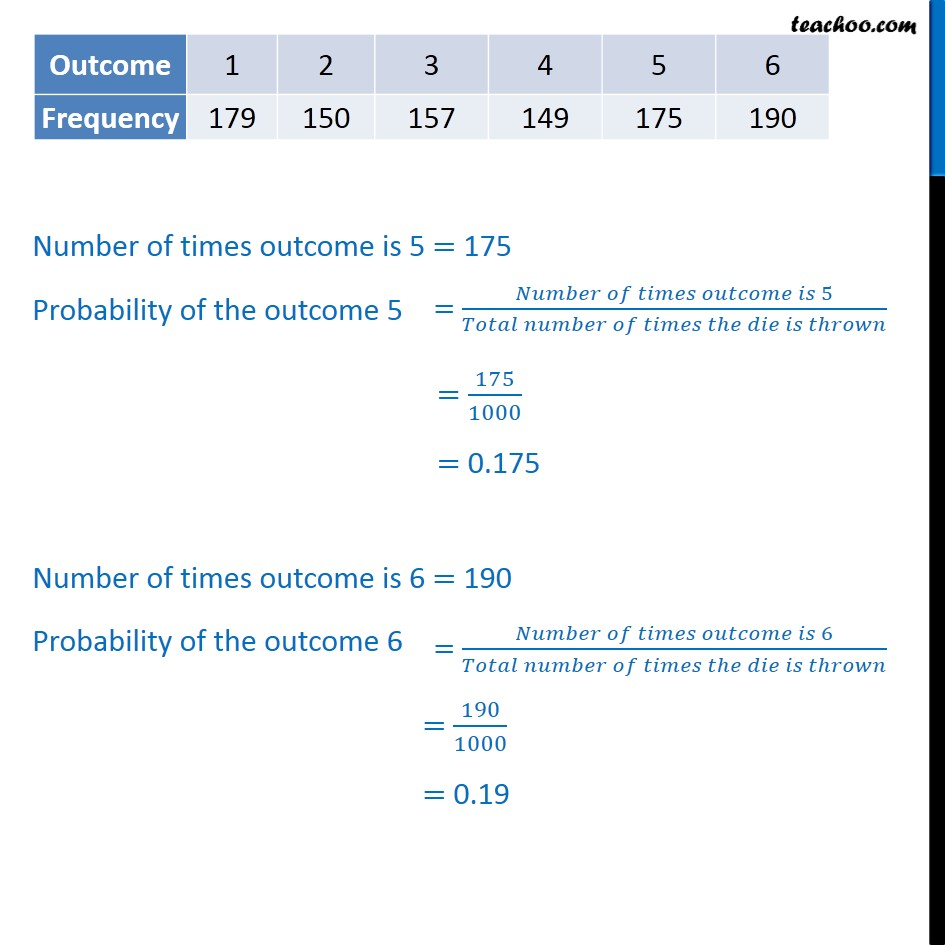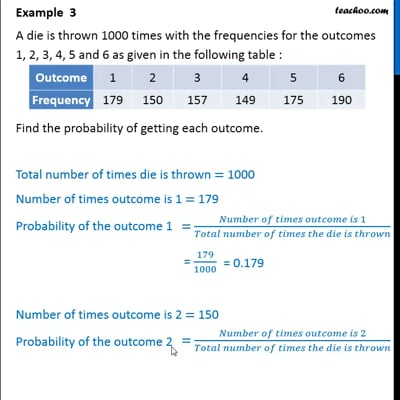Examples

Chapter 15 Class 9 Probability (Term 2)
Serial order wiseThis video is only available for Teachoo black users

### Transcript

Example 3 A die is thrown 1000 times with the frequencies for the outcomes 1, 2, 3, 4, 5 and 6 as given in the following table : Find the probability of getting each outcome. Total number of times die is thrown = 1000 Number of times outcome is 1 = 179 Probability of the outcome 1 = 179/1000 Number of times outcome is 2 = 150 Probability of the outcome 2 = 150/1000 Number of times outcome is 3 = 157 Probability of the outcome 3 = 157/1000 = 0.157 Number of times outcome is 4 = 149 Probability of the outcome 4 = 149/1000 = 0.149 Number of times outcome is 5 = 175 Probability of the outcome 5 = 175/1000 = 0.175 Number of times outcome is 6 = 190 Probability of the outcome 6 = 190/1000 = 0.19